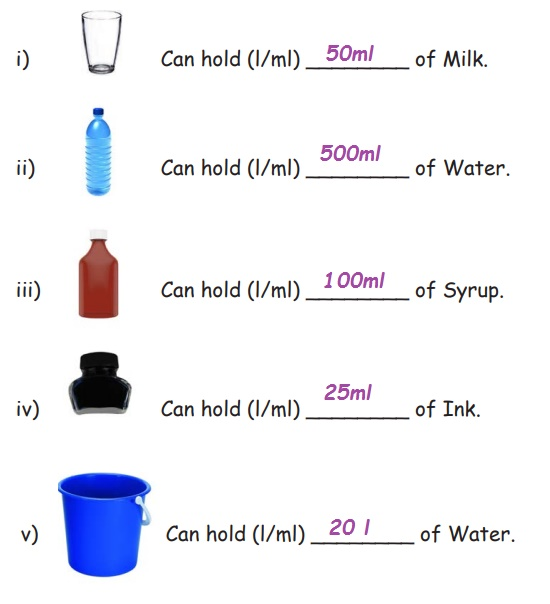Home | | Maths 4th Std | Exercise 3.4 (Estimation of the volume of a liquid contained in a vessel)

# Exercise 3.4 (Estimation of the volume of a liquid contained in a vessel)

Text Book Back Exercises Questions with Answers, Solution : 4th Maths : Term 3 Unit 3 : Measurements : Exercise 3.4 (Estimation of the volume of a liquid contained in a vessel) : Estimate how much liquid each object can hold. (Hint: 500 ml, 100 ml, 50 ml, 25 ml, 20 ml)

Exercise 3.4

1) Estimate how much liquid each object can hold. (Hint: 500 ml, 100 ml, 50 ml, 25 ml, 20 ml)i) Tumbler Can hold (l/ml) 50ml of Milk.

ii) Water Bottle Can hold (l/ml) 500ml of Water.

iii) Syrup Bottle Can hold (l/ml) 100ml of Syrup.

iv) Ink Bottle Can hold (l/ml) 25ml of Ink.

v) Bucket Can hold (l/ml) 20ml of Water.

Tags : Measurements | Term 3 Chapter 3 | 4th Maths , 4th Maths : Term 3 Unit 3 : Measurements
Study Material, Lecturing Notes, Assignment, Reference, Wiki description explanation, brief detail
4th Maths : Term 3 Unit 3 : Measurements : Exercise 3.4 (Estimation of the volume of a liquid contained in a vessel) | Measurements | Term 3 Chapter 3 | 4th Maths